Show TOC

###Actual Cost Distribution in Cost Object Hierarchies#### Use

Actual cost distribution distributes the actual costs collected on a cost object hierarchy to the assigned single objects. This credits the cost object nodes and debits the orders (product cost collectors or manufacturing orders ) with these costs.

#### Integration

You can use the Schedule Manager to assist you in performing the period-end closing activities. For more information, refer to the following sections:

If you use the Schedule Manager with the flow definition, you can use the multilevel worklist to reprocess the faulty objects. For more information, see the following section:

#### Prerequisites

You specify that distribution is active in Customizing for Product Cost Controlling under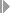Cost Object ControllingProduct Cost by PeriodCost Object HierarchiesDefine Cost Object Categories for Cost Object Hierarchies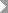.

You can define target cost versions in Customizing through which you establish the calculation base for target cost calculation. You define target cost versions in Customizing for Product Cost by Period underCost Object ControllingProduct Cost by PeriodPeriod-End ClosingVariance CalculationVariance Calculation for Product Cost CollectorsorVariance Calculation for Cost Object HierarchiesDefine Target Cost Versions.

You can also use the following target cost versions in the standard system for actual cost distribution:

• Target cost version 0

• Target cost version 1

• Target cost version 3

If applicable, you carry out functions in the period-end closing activities such as:

• Dynamic template allocation

• Revaluation of activities and business processes at actual prices

#### Features

The distribution process apportions all the actual costs that were assigned to a cost object node.

Actual costs are distributed on the basis of equivalences. The system determines the equivalences during the actual cost distribution process on the basis of target costs.

If you have assigned actual costs directly to an order which is assigned to the cost object hierarchy, these actual costs are not distributed.

#### Cost Object Hierarchy: Actual Cost Distribution on the Basis of Target Costs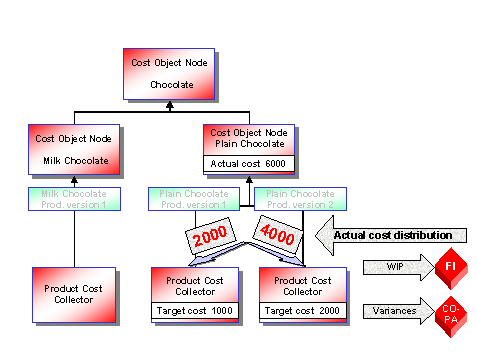( )

The actual costs are distributed on the basis of the target costs calculated from the delivered quantities for each order.

This means that the distributed actual costs are carried by the yield quantity.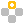Example

Example 1: Actual cost distribution when no scrap was confirmed

In our example, production order 4712 manufactures 100 units of material P-100, and production order 4713 manufactures 100 units of material P-110. No scrap was incurred with either production order. 100 units of yield were confirmed for each production order.

Both production orders are charged with actual costs of USD 100.

Both production orders were credited with DM 100 when the goods receipt was entered.

End of the example.

#### Actual Cost Distribution When No Scrap Was Confirmed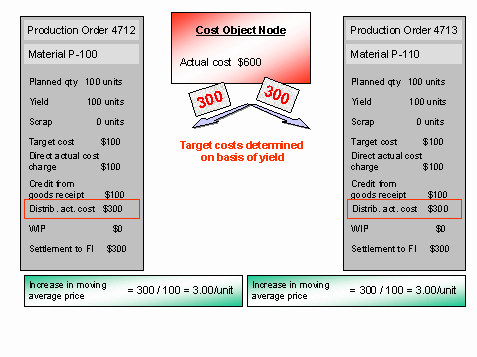( )

To calculate target costs, the system converts the planned values to the yields. In our example, it is assumed that net target costs of USD 1 were calculated for each unit produced. This results in target costs of USD 100 for both production orders. The target costs are in a 1:1 relationship to each other. This results in a distribution ratio of 1/2 to production order 4712 and 1/2 to production order 4713. The actual costs of USD 600 on the superior cost object node are therefore distributed as follows:

USD 300 to production order 4712

USD 300 to production order 4713

The work in process for each production order is zero.

This results in an order balance of USD 300 for production order 4712 that is calculated as follows:

Direct actual cost debit USD 100

Plus distributed actual costs USD 300

Less credit for goods receipt USD 100

Order balance USD 300

The order balance is settled to FI.

The settled balance causes the (statistical) moving average price to increase by USD 3 per unit (Order balance / Yield = 300 / 100).

This results in an order balance of USD 300 for production order 4713 that is calculated as follows:

Direct actual cost debit USD 100

Plus distributed actual costs USD 300

Less credit for goods receipt USD 100

Order balance USD 300

The order balance is settled to FI.

The settled balance causes the moving average price to increase by USD 3 per unit (Order balance / Yield = 300 / 100).

If any scrap arises during the production of particular materials, this together with actual cost distribution will cause the moving average price for the other materials to increase unrealistically.Example

Example 2: Actual cost distribution when scrap was confirmed

In our example, production order 4712 manufactures 100 units of material P-100, and production order 4713 manufactures 100 units of material P-110. For production order 4712, 50 units of yield and 50 units of scrap were confirmed. For production order 4713, 100 units of yield and 0 units of scrap were confirmed.

Both production orders are charged with actual costs of USD 100. Production order 4712 was credited with USD 50 for the goods receipt, and production order 4713 with USD 100.

End of the example.

#### Actual Cost Distribution When Scrap Was Confirmed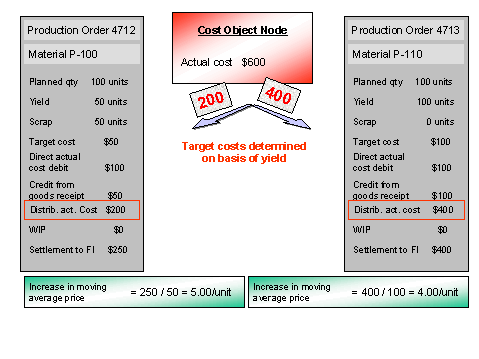( )

To calculate target costs, the system converts the planned values to the yields. It is again assumed that net target costs of USD 1 were calculated for each unit produced. This results in target costs of USD 50 for production order 4712 and target costs of USD 100 for production order 4713. The target costs are in a 1:2 relationship to each other. This results in a distribution ratio of 1/3 to production order 4712 and 2/3 to production order 4713. The actual costs of USD 600 on the superior cost object node are therefore distributed as follows:

USD 200 to production order 4712

USD 400 to production order 4713

The work in process for each production order is zero.

This results in an order balance of USD 250 for production order 4712 that is calculated as follows:

Direct actual cost debit USD 100

Plus distributed actual costs USD 200

Less credit for goods receipt USD 50

Order balance USD 250

The order balance is settled to FI.

The settled balance causes the moving average price to increase by USD 5 per unit (Order balance / Yield = 250 / 50).

This results in an order balance of USD 400 for production order 4713 that is calculated as follows:

Direct actual cost debit USD 100

Plus distributed actual costs USD 400

Less credit for goods receipt USD 100

Order balance USD 400

The order balance is settled to FI.

The settled balance causes the moving average price to increase by USD 4 per unit (Order balance / Yield = 400 / 100).

In example 2, an additional amount of USD 100 is distributed to production order 4713 even though the situation on the production order itself has not changed. The additional charge is due to the fact that production order 4712 had a lower yield and therefore fewer actual costs are distributed to it. The higher order balance of production order 4713 will come into play especially when the price indicator of material P-110 is set to V. However, SAP recommends against using price indicator V for internally manufactured materials (see also: Basic Decisions in Cost Object Controlling ).

Improving Performance

You can use parallel processing to reduce the runtime of the actual cost distribution process.

• If you perform actual cost distribution outside the Schedule Manager , specify a server group for background processing.

• If you perform actual cost distribution using the Schedule Manager , set the Parallel processing indicator in the selection screen of the program when you create the job variant. An entry field then appears in which you enter the server group.

Schedule Manager: Multilevel Worklist

You can view and edit the objects with errors that were processed with the individual functions. You use the multilevel worklist for this purpose. The multilevel worklist enables you to edit the faulty objects of an individual processing step (that is, a single function) or of an entire processing step sequence.

A prerequisite for the multilevel worklist is that you are using the Schedule Manager in conjunction with a flow definition.

See: Integration in this section.

Transfer Prices: Multiple Valuation Views

In actual cost distribution for cost object hierarchies, you can update the data in multiple valuation views. For more information, see the following section:

User-Defined Error Management

You can influence the messages output in the log of actual cost distribution by using user-defined error management .

#### Activities

The following system activities take place during distribution:

1. The system calculates the target cost for each cost element or cost element and origin group or origin depending on the cost estimate for the different target cost versions and the quantity delivered in the period (yield).

Target costs are calculated as follows:

• Costs that vary with the lot size are divided by the lot size of the standard cost estimate and multiplied by the quantity delivered in the period.

• Costs that do not vary with the lot size (such as setup costs) are used directly as target costs.

Target costs are calculated on the basis of a target cost version that you define in Customizing for Product Cost Controlling .

The target costs for a cost object hierarchy can be calculated with the following target cost versions:

• Target cost version 0

• Target cost version 1

• Target cost version 3

1. The system creates equivalences between the assigned orders using the target costs for the relevant cost element.

2. The system distributes to the orders the actual costs collected at the level of the hierarchy in accordance with these equivalences, and updates the actual costs on the orders. The single objects assigned to the cost object hierarchy are debited with actual costs. The cost object node (the sender of the distributed costs) is credited accordingly.

3. After distribution, you settle all actual costs (that is, the actual costs that were assigned directly to the single objects plus the distributed actual costs).

This enables you to see the actual costs in the period for each product, and calculate a moving average price for the materials being produced.